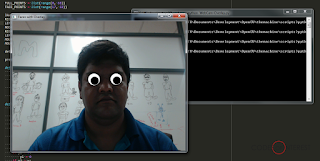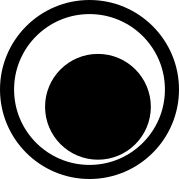# Snapchat like Image Overlays with Dlib, OpenCV, and Python

You're probably familiar with Snapchat, and it's filters feature where you can put some cool and funny image overlays on your face images. As computer vision enthusiasts, we typically look at applications like these, and try to understand how it's done, and whether we can build something similar.

It turns out, we can make our own application with Snapchat like image overlays using Python, OpenCV, and Dlib.Snapchat like Image Overlays with Dlib, OpenCV, and Python

So, how do we build it?
1. We'll first load the Webcam feed using OpenCV.
2. We'll load an image (in our example, and image for the 'eye') to be used as the overlay.
3. Use Dlib's face detection to localize the faces, and then use facial landmarks to find where the eyes are.
4. Calculate the size and the position of the overlay for each eye.
5. Finally, place the overlay image over each eye, resized to the correct size.

Let's start.

`````` import numpy as np
import cv2
import dlib
from scipy.spatial import distance as dist
from scipy.spatial import ConvexHull
``````

Apart from OpenCV and Dlib, we load two methods from the scipy.spatial package, that'll help us with distance and size calculations.

Next, we setup the parameters for the Dlib Face Detector and Face Landmark detector. We also initialize the arrays that help us extract individual face landmarks out of the 68 landmarks which Dlib returns. (see Extracting individual Facial Features from Dlib Face Landmarks).

`````` PREDICTOR_PATH = "path/to/your/shape_predictor_68_face_landmarks.dat"

FULL_POINTS = list(range(0, 68))
FACE_POINTS = list(range(17, 68))

JAWLINE_POINTS = list(range(0, 17))
RIGHT_EYEBROW_POINTS = list(range(17, 22))
LEFT_EYEBROW_POINTS = list(range(22, 27))
NOSE_POINTS = list(range(27, 36))
RIGHT_EYE_POINTS = list(range(36, 42))
LEFT_EYE_POINTS = list(range(42, 48))
MOUTH_OUTLINE_POINTS = list(range(48, 61))
MOUTH_INNER_POINTS = list(range(61, 68))

detector = dlib.get_frontal_face_detector()

predictor = dlib.shape_predictor(PREDICTOR_PATH)
``````

Next, we'll load the image for the overlay.

I'll be using the following image as the overlay for the eyes,The overlay image for the eyes
Notice that the image is a PNG, with a transparent background. The transparency is important, as we only want to place the eye, without a white box around it.

`````` #---------------------------------------------------------
# Load and pre-process the eye-overlay
#---------------------------------------------------------
# Load the image to be used as our overlay

# Create the mask from the overlay image

# Create the inverted mask for the overlay image

# Convert the overlay image image to BGR
# and save the original image size
imgEye = imgEye[:,:,0:3]
origEyeHeight, origEyeWidth = imgEye.shape[:2]
``````

Notice the '-1' parameter in cv2.imread. It's telling OpenCV to load the 'Alpha Channel' (a.k.a. the transparency channel) of the image, along with the BGR channels.
We take the alpha channel and create a mask from it. We create an inverse of the mark also, which will be used to define the pixels outside of the eye overlay.
We then convert the overlay image back to a regular BGR image, removing the alpha channel.

We now start capturing the frames from the Webcam, detects the faces from the frame, and detect the face landmarks. We extract out the landmarks for the left and right eyes separately from the landmark array.

`````` # Start capturing the WebCam
video_capture = cv2.VideoCapture(0)

while True:

if ret:
gray = cv2.cvtColor(frame, cv2.COLOR_BGR2GRAY)

rects = detector(gray, 0)

for rect in rects:
x = rect.left()
y = rect.top()
x1 = rect.right()
y1 = rect.bottom()

landmarks = np.matrix([[p.x, p.y] for p in predictor(frame, rect).parts()])

left_eye = landmarks[LEFT_EYE_POINTS]
right_eye = landmarks[RIGHT_EYE_POINTS]
``````

In order to place the overlay on to the eyes of the face image, we need to find the size and the center of each of the eyes. We define a function in order to calculate them,

`````` def eye_size(eye):
eyeWidth = dist.euclidean(eye, eye)
hull = ConvexHull(eye)
eyeCenter = np.mean(eye[hull.vertices, :], axis=0)

eyeCenter = eyeCenter.astype(int)

return int(eyeWidth), eyeCenter
``````

We use the euclidean function to calculate the width of the eye, and the ConvexHull function to calculate the center.

We pass each of the eyes separately to get their sizes individually,

`````` leftEyeSize, leftEyeCenter = eye_size(left_eye)
rightEyeSize, rightEyeCenter = eye_size(right_eye)
``````

Now it's time to place the overlay on to the face image. We define the place_eye function for that,

`````` def place_eye(frame, eyeCenter, eyeSize):
eyeSize = int(eyeSize * 1.5)

x1 = int(eyeCenter[0,0] - (eyeSize/2))
x2 = int(eyeCenter[0,0] + (eyeSize/2))
y1 = int(eyeCenter[0,1] - (eyeSize/2))
y2 = int(eyeCenter[0,1] + (eyeSize/2))

h, w = frame.shape[:2]

# check for clipping
if x1 < 0:
x1 = 0
if y1 < 0:
y1 = 0
if x2 > w:
x2 = w
if y2 > h:
y2 = h

# re-calculate the size to avoid clipping
eyeOverlayWidth = x2 - x1
eyeOverlayHeight = y2 - y1

# calculate the masks for the overlay
eyeOverlay = cv2.resize(imgEye, (eyeOverlayWidth,eyeOverlayHeight), interpolation = cv2.INTER_AREA)

# take ROI for the verlay from background, equal to size of the overlay image
roi = frame[y1:y2, x1:x2]

# roi_bg contains the original image only where the overlay is not, in the region that is the size of the overlay.

# roi_fg contains the image pixels of the overlay only where the overlay should be

# join the roi_bg and roi_fg

# place the joined image, saved to dst back over the original image
frame[y1:y2, x1:x2] = dst
``````

Here, we're calculating the size for the overlay based on the eye size and position.

We also need to check for clipping. Otherwise, you'll be getting an error like the following when you try to calculate a mask that has pixels outside of the image frame.

`````` OpenCV Error: Assertion failed ((mtype == CV_8U || mtype == CV_8S) && _mask.same
Size(*psrc1)) in cv::binary_op, file C:\bld\opencv_1492084805480\work\opencv-3.2
.0\modules\core\src\arithm.cpp, line 241
Traceback (most recent call last):
File "WebCam-Overlay.py", line 135, in <module>
place_eye(frame, leftEyeCenter, leftEyeSize)
File "WebCam-Overlay.py", line 51, in place_eye
cv2.error: C:\bld\opencv_1492084805480\work\opencv-3.2.0\modules\core\src\arithm
.cpp:241: error: (-215) (mtype == CV_8U || mtype == CV_8S) && _mask.sameSize(*ps
rc1) in function cv::binary_op
``````

What we are basically doing here is to calculate the size of the overlay, get a box of pixels of that size out of the face image around the position of where the overlay should go, substitute the pixels of that extracted box with the pixels from the overlay image excluding the transparent pixels (using the masks we calculate), and finally put the substituted box of pixels back in to the face image.

We need to do this for each eye individually,

`````` place_eye(frame, leftEyeCenter, leftEyeSize)
place_eye(frame, rightEyeCenter, rightEyeSize)
``````

Finally, we just need to show the resulting frame,

`````` cv2.imshow("Faces with Overlay", frame)
``````

And here's the result,The image overlay working

Since we calculate the size of each eye individually for the overlay, they show up correctly when you turn your head,The overlays resize correctly when you turn tour head

And, it even works when wearing glasses,The overlays works with glasses also

Check the video to see the image overlays in action,

Here's the full code for your convenience,

`````` import numpy as np
import cv2
import dlib
from scipy.spatial import distance as dist
from scipy.spatial import ConvexHull

PREDICTOR_PATH = "path/to/your/shape_predictor_68_face_landmarks.dat"

FULL_POINTS = list(range(0, 68))
FACE_POINTS = list(range(17, 68))

JAWLINE_POINTS = list(range(0, 17))
RIGHT_EYEBROW_POINTS = list(range(17, 22))
LEFT_EYEBROW_POINTS = list(range(22, 27))
NOSE_POINTS = list(range(27, 36))
RIGHT_EYE_POINTS = list(range(36, 42))
LEFT_EYE_POINTS = list(range(42, 48))
MOUTH_OUTLINE_POINTS = list(range(48, 61))
MOUTH_INNER_POINTS = list(range(61, 68))

detector = dlib.get_frontal_face_detector()

predictor = dlib.shape_predictor(PREDICTOR_PATH)

def eye_size(eye):
eyeWidth = dist.euclidean(eye, eye)
hull = ConvexHull(eye)
eyeCenter = np.mean(eye[hull.vertices, :], axis=0)

eyeCenter = eyeCenter.astype(int)

return int(eyeWidth), eyeCenter

def place_eye(frame, eyeCenter, eyeSize):
eyeSize = int(eyeSize * 1.5)

x1 = int(eyeCenter[0,0] - (eyeSize/2))
x2 = int(eyeCenter[0,0] + (eyeSize/2))
y1 = int(eyeCenter[0,1] - (eyeSize/2))
y2 = int(eyeCenter[0,1] + (eyeSize/2))

h, w = frame.shape[:2]

# check for clipping
if x1 < 0:
x1 = 0
if y1 < 0:
y1 = 0
if x2 > w:
x2 = w
if y2 > h:
y2 = h

# re-calculate the size to avoid clipping
eyeOverlayWidth = x2 - x1
eyeOverlayHeight = y2 - y1

# calculate the masks for the overlay
eyeOverlay = cv2.resize(imgEye, (eyeOverlayWidth,eyeOverlayHeight), interpolation = cv2.INTER_AREA)

# take ROI for the verlay from background, equal to size of the overlay image
roi = frame[y1:y2, x1:x2]

# roi_bg contains the original image only where the overlay is not, in the region that is the size of the overlay.

# roi_fg contains the image pixels of the overlay only where the overlay should be

# join the roi_bg and roi_fg

# place the joined image, saved to dst back over the original image
frame[y1:y2, x1:x2] = dst

#---------------------------------------------------------
# Load and pre-process the eye-overlay
#---------------------------------------------------------
# Load the image to be used as our overlay

# Create the mask from the overlay image

# Create the inverted mask for the overlay image

# Convert the overlay image image to BGR
# and save the original image size
imgEye = imgEye[:,:,0:3]
origEyeHeight, origEyeWidth = imgEye.shape[:2]

# Start capturing the WebCam
video_capture = cv2.VideoCapture(0)

while True:

if ret:
gray = cv2.cvtColor(frame, cv2.COLOR_BGR2GRAY)

rects = detector(gray, 0)

for rect in rects:
x = rect.left()
y = rect.top()
x1 = rect.right()
y1 = rect.bottom()

landmarks = np.matrix([[p.x, p.y] for p in predictor(frame, rect).parts()])

left_eye = landmarks[LEFT_EYE_POINTS]
right_eye = landmarks[RIGHT_EYE_POINTS]

# cv2.rectangle(frame, (x, y), (x1, y1), (0, 255, 0), 2)

leftEyeSize, leftEyeCenter = eye_size(left_eye)
rightEyeSize, rightEyeCenter = eye_size(right_eye)

place_eye(frame, leftEyeCenter, leftEyeSize)
place_eye(frame, rightEyeCenter, rightEyeSize)

cv2.imshow("Faces with Overlay", frame)

ch = 0xFF & cv2.waitKey(1)

if ch == ord('q'):
break

cv2.destroyAllWindows()

``````

We only tried an overlay for the eyes here. But using the same techniques, you would be able to create overlays for anything. So, unleash your creativity, and what you can come up with.

Related posts:
Extracting individual Facial Features from Dlib Face Landmarks

https://sublimerobots.com/2015/02/dancing-mustaches/

Build Deeper: The Path to Deep Learning

Learn the bleeding edge of AI in the most practical way: By getting hands-on with Python, TensorFlow, Keras, and OpenCV. Go a little deeper...